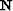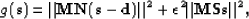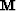Next: Adaptive subtraction Up: Multiple subtraction Previous: Multiple subtraction

## Pattern-based approach

The pattern-based approach separates noise and signal according to their multivariate spectra. In this Chapter, the patterns are approximated with 3-D prediction-error filters (PEFs). Callingthe PEFs for the multiples estimated from the multiple model with SRMP,the PEFs for the signal estimated from the Spitz approximation Spitz (1999) andthe primaries, the objective function to minimize becomes(70)
whereis a masking operator that preserves the signal where no multiples are present. The signal is estimated iteratively with a conjugate gradient method, and not with the Huber norm of Chapter. In few words, the Spitz approximation consists in (1) convolving the noise PEFs N with the data d, and (2) estimating the signal PEFs S from the convolution result. As demonstrated by Abma (1995), the least-squares inverse ofin equation () is an optimal Wiener filter. Similar to Chapter, the dataset is divided into macro-patches of 50 consecutive shots with an overlap of 5 shots before the separation. Then the macro-patches are reassembled to form the final result.Next: Adaptive subtraction Up: Multiple subtraction Previous: Multiple subtraction
Stanford Exploration Project
5/5/2005